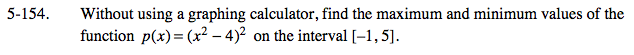### Home > CALC > Chapter 5 > Lesson 5.5.1 > Problem5-154

5-154.Candidates for global maxima and global minima exist where the derivative equals 0 AND where the derivative does not exist.... Notice that this is a function that has a closed domain. That means that we already know two candidates for global max and min: the endpoints.

Evaluate the endpoints:
p(−1) = 9
p(5) = 21² = 441
These might be the global min and max, respectively. We still need to check the other candidates.

Find additional candidates by setting the derivative equal to zero, and solving for x.
p'(x) = 4x(x² − 4)
0 = 4x(x − 2)(x + 2)
x = 0, x = 2 and x = −2 are candidates...
but eliminate x = −2 because it is not within the given domain.

Evaluate the remaining candidates: p(0) = 16, p(2) = 0 and compare to the endpoint candidates.

Recall that maxima and minima are y-values.
Therefore the global maximum is 441 and the global minimum is 0.It should also be noted that 16 is a local maximum.
Confirm this by sketching p(x) on your graphing calculator.